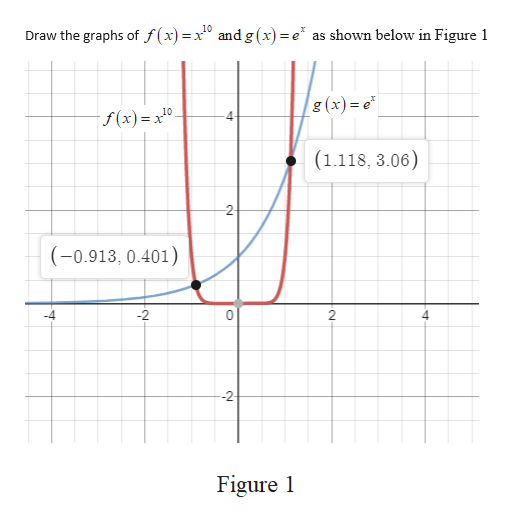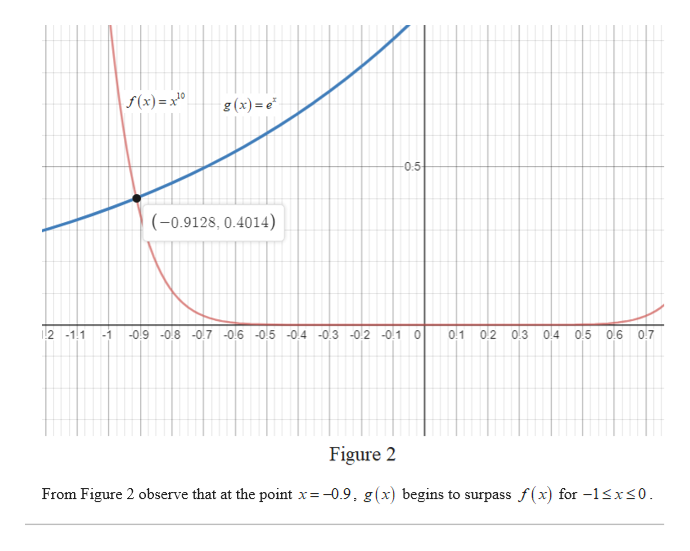# 4)  Compare the function f(x)=x^10 and g(x)=e^x by graphing both function in serveral viewing window on Maple .When dose the graph of g(x) Finally surpass the graph of f(x). Note:- Solve it using Maple

Question
38 views

4)  Compare the function f(x)=x^10 and g(x)=e^x by graphing both function in serveral viewing window on Maple .When dose the graph of g(x) Finally surpass the graph of f(x).

Note:- Solve it using Maple

check_circle

Step 1help_outlineImage TranscriptioncloseDraw the graphs of f(x) x 10 andg (x) =e as shown below in Figure 1 g(x)= e f(x)= x10 4- (1.118, 3.06) 2- (-0.913, 0.401) 2 Figure et fullscreen
Step 2
Step 3help_outlineImage Transcriptionclosef(x) = x10 g (x)= e 0.5 (0.9128, 0.4014) 01 02 0.3 o.4 0.5 2 -1.1 -1 -0.9 -08 -07 -06 05 -04 -03 -0.2 -01 0 0.6 0.7 Figure 2 From Figure 2 observe that at the point x=-0.9, g(x) begins to surpass f(x) for -13xs0 16 fullscreen

### Want to see the full answer?

See Solution

#### Want to see this answer and more?

Solutions are written by subject experts who are available 24/7. Questions are typically answered within 1 hour.*

See Solution
*Response times may vary by subject and question.
Tagged in

### Calculus